# Math Is Fun Forum

Discussion about math, puzzles, games and fun.   Useful symbols: ÷ × ½ √ ∞ ≠ ≤ ≥ ≈ ⇒ ± ∈ Δ θ ∴ ∑ ∫  π  -¹ ² ³ °

You are not logged in.

## #1 2010-05-20 04:45:17

ZHero
Real MemberRegistered: 2008-06-08
Posts: 1,889

### Perfect Squares..

I just wanted to find all the Perfect Squares (except 0, 1, 4 and 9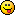) such that if their Unit's and Ten's digits are interchanged, the resulting number is again a Perfect Square!

I know a few

.

How can I find ALL SUCH Perfect Squares??

If two or more thoughts intersect, there has to be a point!

Offline

## #2 2010-05-20 05:32:48

gurthbruins
Member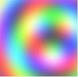Registered: 2010-05-09
Posts: 157

### Re: Perfect Squares..

Do you do any computer programming? If so you could get your computer to churn them out in quite a large quantity... and then maybe spot some patterns... I think people interested in maths should be a bit computer literate - I have found even professional mathematicians strangely resistant to computer science.

It's the activity of the intelligence above all that gives charm to existence.

Offline

## #3 2010-05-20 06:26:37

ZHero
Real MemberRegistered: 2008-06-08
Posts: 1,889

### Re: Perfect Squares..

I learned C when in Second year of my Four year Engg course and my sis (who has command over more than 7 different programming languages) tried to teach me CPP some years back.
I still have a fair idea of C but 've forgottenmost of the other things coz of not using them for 3 years!
Although I like your proposition yet i'd like to know how to work it out using Algebra?If two or more thoughts intersect, there has to be a point!

Offline

## #4 2010-05-20 07:23:35

gurthbruins
MemberRegistered: 2010-05-09
Posts: 157

### Re: Perfect Squares..

- not a job that appeals to me, I regret...

It's the activity of the intelligence above all that gives charm to existence.

Offline

## #5 2010-05-20 09:07:08

TheDude
Member
Registered: 2007-10-23
Posts: 361

### Re: Perfect Squares..

Well, you can narrow your search fairly easily.  There are two ways for your attribute to hold true: the two squares must have a difference of less than 100 or the unit's and ten's digits are equal.  For the first method there are only a finite number of possibilities since the distance between consecutive squares strictly increases.  Specifically, only the squares of 4 through 50 are eligible, and it's a pretty simple matter to brute force.  As it happens there is exactly one such pair of squares - 13 & 14.

For the second method you should note that in multiplication the final two digits of the product are decided solely by the final two digits of the multiplicands.  Again you can simply brute force numbers from 4 to 99 and check by hand.  For squares found using this method you can add any multiple of 100 and it will still work.  Aside from the obvious multiples of 10, the squares that fall under this category are 12, 38, 62, and 88.

In short, the numbers you are looking for are the squares of 10, 12, 13, 14, 20, 30, 38, 40, 50, 60, 62, 70, 80, 88, and 90, and any multiple of 100 plus any of those numbers except 13 and 14.

Wrap it in bacon

Offline

## #6 2010-05-20 19:52:03

ZHero
Real MemberRegistered: 2008-06-08
Posts: 1,889

### Re: Perfect Squares..

TheDude wrote:

Specifically, only the squares of 4 through 50 are eligible, and it's a pretty simple matter to brute force.  As it happens there is exactly one such pair of squares - 13 & 14.

That's really tricky but now i got it (after checking the maximum difference possible between two numbers who's Last Two digits are same, may be in different orders)!!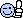TheDude wrote:

In short, the numbers you are looking for are the squares of 10, 12, 13, 14, 20, 30, 38, 40, 50, 60, 62, 70, 80, 88, and 90, and any multiple of 100 plus any of those numbers except 13 and 14.

Your last sentence again holds a Lot of Information! Thx!!

TheDude wrote:

For the second method you should note that in multiplication the final two digits of the product are decided solely by the final two digits of the multiplicands.  Again you can simply brute force numbers from 4 to 99 and check by hand.  For squares found using this method you can add any multiple of 100 and it will still work.  Aside from the obvious multiples of 10, the squares that fall under this category are 12, 38, 62, and 88.

I'll try and see if some sort of Algebraic method can help give those numbersEarlier i was wondering if big perfect square xxxxxxxxxab exists such that xxxxxxxxxba is also perfect square (a,b≠0). Now i see that such a number can't be any greater than 7744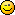Thanks dudeIf two or more thoughts intersect, there has to be a point!

Offline

## #7 2010-05-20 19:54:32

ZHero
Real MemberRegistered: 2008-06-08
Posts: 1,889

### Re: Perfect Squares..

gurthbruins wrote:

- not a job that appeals to me, I regret...

........sigh......

old fermat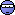If two or more thoughts intersect, there has to be a point!

Offline

## #8 2010-05-21 00:34:09

TheDude
Member
Registered: 2007-10-23
Posts: 361

### Re: Perfect Squares..

ZHero wrote:

Earlier i was wondering if big perfect square xxxxxxxxxab exists such that xxxxxxxxxba is also perfect square (a,b≠0). Now i see that such a number can't be any greater than 7744No, there are infinitely many such squares.  Specifically, the square of 100z + {12, 38, 62, 88} for any non-negative integer z will end in xxxxxxxxx44.  However, there are only 2 examples of perfect squares with this attribute that don't end in either xxxxxxx00 or xxxxxxx44 - 169 and 196.

Wrap it in bacon

Offline

## #9 2010-05-21 00:40:00

gurthbruins
MemberRegistered: 2010-05-09
Posts: 157

### Re: Perfect Squares..

ZHero wrote:
gurthbruins wrote:

- not a job that appeals to me, I regret...

........sigh......

old fermat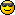I was and am always lazy : that's a great motivator, I've never regretted it.It's the activity of the intelligence above all that gives charm to existence.

Offline

## #10 2010-05-21 00:47:04

ZHero
Real MemberRegistered: 2008-06-08
Posts: 1,889

### Re: Perfect Squares..

TheDude wrote:

No, there are infinitely many such squares.  Specifically, the square of 100z + {12, 38, 62, 88} for any non-negative integer z will end in xxxxxxxxx44.

you mean as in (1100+12)[sup]2[/sup] = 1236544

xxxxxxxab and (a,b)≠(00, 44)Thanks Again!Last edited by ZHero (2010-05-21 00:48:27)

If two or more thoughts intersect, there has to be a point!

Offline

## #11 2010-05-26 17:49:04

ZHero
Real MemberRegistered: 2008-06-08
Posts: 1,889

### Re: Perfect Squares..

Hmmm...
Let (100y+10a+b) = M[sup]2[/sup] ; ∀ y ∈ Z[sup]+[/sup] and {a, b} ∈ {0, 1, 2, 3, 4, 5, 6, 7, 8, 9}

We want (100y+10b+a) to be a perfect square too.
Let (100y+10b+a) = N[sup]2[/sup]

We can see that...
M[sup]2[/sup] - N[sup]2[/sup] = 9(a-b)
or, M[sup]2[/sup] - N[sup]2[/sup] = 9k ; k ∈ {0, 1, 2, 3, 4, 5, 6, 7, 8, 9}

The question is, in fact, looking for two numbers M[sup]2[/sup] and N[sup]2[/sup] such that their difference is a multiple of 9 i.e. ∈ {0, 9, 18, 27, 36, 45, 54, 63, 81}

M[sup]2[/sup] - N[sup]2[/sup] = 9k
(M+N)(M-N) = 9k
since {M, N} ∈ Z[sup]+[/sup]
∴ (M+N) and (M-N) must be either both Even or both Odd factors of 9k.
Hence, only possible values of 9k are {0, 9, 27, 36, 45, 63, 81}

for (M+N)(M-N) = 9 = 9×1 M=5 and N=4

for (M+N)(M-N) = 27 = 9×3 M=6 and N=3
for (M+N)(M-N) = 27 = 27×1 M=14 and N=13

for (M+N)(M-N) = 36 = 18×2 M=10 and N=8

for (M+N)(M-N) = 45 = 9×5 M=10 and N=8
for (M+N)(M-N) = 45 = 45×1 M=23 and N=22

for (M+N)(M-N) = 63 = 9×7 M=8 and N=1
for (M+N)(M-N) = 63 = 63×1 M=32 and N=31

for (M+N)(M-N) = 81 = 9×9 M=9 and N=0
for (M+N)(M-N) = 81 = 27×3 M=15 and N=12
for (M+N)(M-N) = 81 = 81×1 M=41 and N=40

for the Special Case of ZHero(M+N)(M-N) = 0 M=N

I referred WikiPedia.

WikiPedia wrote:

A square number can only end with digits 00,1,4,6,9, or 25 in base 10, as follows:

1. If the last digit of a number is 0, its square ends in 00 and the preceding digits must also form a square.

2. If the last digit of a number is 1 or 9, its square ends in 1 and the number formed by its preceding digits must be divisible by four.

3. If the last digit of a number is 2 or 8, its square ends in 4 and the preceding digit must be even.

4. If the last digit of a number is 3 or 7, its square ends in 9 and the number formed by its preceding digits must be divisible by four.

5. If the last digit of a number is 4 or 6, its square ends in 6 and the preceding digit must be odd.

6. If the last digit of a number is 5, its square ends in 25 and the preceding digits must be 0, 2, 06, or 56.

It can be seen that points 2. and 6. are not possible and for the remaining possibilities, only 00 and 44 (since M=N) are the possibilities for the last two digits!!!

Points 4. and 5. have been covered in the M=14 and N=13If two or more thoughts intersect, there has to be a point!

Offline